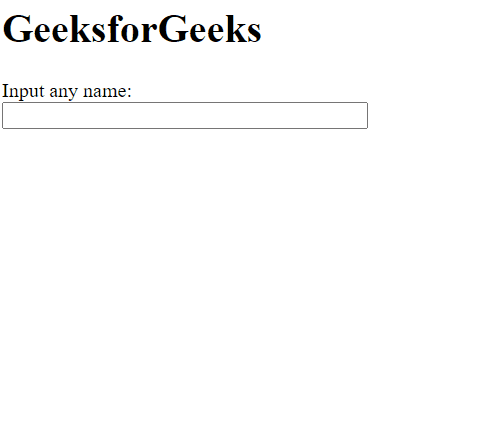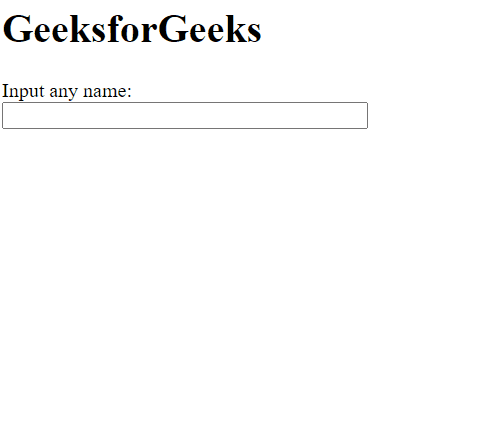Related Articles
script.aculo.us Autocompleter.Local partialSearch Option
• Last Updated : 20 Nov, 2020

The script.aculo.us library is a cross-browser library that aims to improving the user interface of a website. The Autocompleter can be used to providing auto-completion support for text fields in the webpage. The local Autocompleter is used when auto-completion options are given as an array to the Autocompleter method to display as the choices.

The Autocompleter.Local partialSearch option is used to set whether the autocompleter will match the entered text only at the beginning of strings in the given array. A value of true means that it will only match text at the beginning of any word in the strings of the array, whereas a value of false will match the text at any position of the word.

Syntax:

```{ partialSearch: boolean }
```

Values:

• boolean: This is a boolean value that specifies whether the text would be matched only at the beginning of the strings. The default value is false.

Example 1: In this example, we have set the partialSearch option to false, so it will search only from the starting of the string.

## HTML

 ```<``html``>`` ` `<``head``>``    ````    ``<``script` `type``=``"text/javascript"` `        ``src``=``"prototype.js"``>``    ```` ` `    ``<``script` `type``=``"text/javascript"` `src``=``"scriptaculous.js?load = effects,controls"``>``    `````` ` `<``body``>``    ``<``h1``>GeeksforGeeks`` ` `    ``<``label` `for``=``"GeeksforGeeks"``>``        ``Input any name:``    ````     ` `    ``<``br` `/>``    ``<``input` `id``=``"GeeksforGeeks"` `autocomplete``=``"off"``        ``size``=``"40"` `type``=``"text"` `value``=``""` `/>`` ` `    ``<``div` `class``=``"autocomplete"` `id``=``"names"``        ``style``=``"display:none"``>``    ```` ` `    ``<``script` `type``=``"text/javascript"``>`` ` `        ``// Array to be used as choices``        ``var names = [``            ``'Ab gfg',``            ``'Abc gfg',``            ``'Abcd gfg',``            ``'Abcde gfg',``            ``'Abcdef gfg',``            ``'Abcdefg gfg',``            ``'Abcdefgh gfg'``        ``];`` ` `        ``// Initialize the Autocompleter``        ``new Autocompleter.Local('GeeksforGeeks',``            ``'names', names, {`` ` `            ``// Specify whether only the ``            ``// beginning of the strings``            ``// would be searched``            ``partialSearch: false``        ``});``    `````` ` ``

Output:Example 2: In this example, we have set the partialSearch option to true, so it will search from anywhere in the string.

## HTML

 ```<``html``>`` ` `<``head``>``    ````    ``<``script` `type``=``"text/javascript"` `        ``src``=``"prototype.js"``>``    ```` ` `    ``<``script` `type``=``"text/javascript"` `src``=``"scriptaculous.js?load = effects,controls"``>``    `````` ` `<``body``>``    ``<``h1``>GeeksforGeeks`` ` `    ``<``label` `for``=``"GeeksforGeeks"``>``        ``Input any name:``    ````     ` `    ``<``br` `/>``    ``<``input` `id``=``"GeeksforGeeks"` `autocomplete``=``"off"``        ``size``=``"40"` `type``=``"text"` `value``=``""` `/>`` ` `    ``<``div` `class``=``"autocomplete"` `id``=``"names"``        ``style``=``"display:none"``>``    ```` ` `    ``<``script` `type``=``"text/javascript"``>`` ` `        ``// Array to be used as choices``        ``var names = [``            ``'Ab gfg',``            ``'Abc gfg',``            ``'Abcd gfg',``            ``'Abcde gfg',``            ``'Abcdef gfg',``            ``'Abcdefg gfg',``            ``'Abcdefgh gfg'``        ``];`` ` `        ``// Initialize the Autocompleter``        ``new Autocompleter.Local('GeeksforGeeks',``            ``'names', names, {`` ` `            ``// Specify whether only the beginning``            ``// of the strings would be searched``            ``partialSearch: true``        ``});``    `````` ` ``

Output:My Personal Notes arrow_drop_up